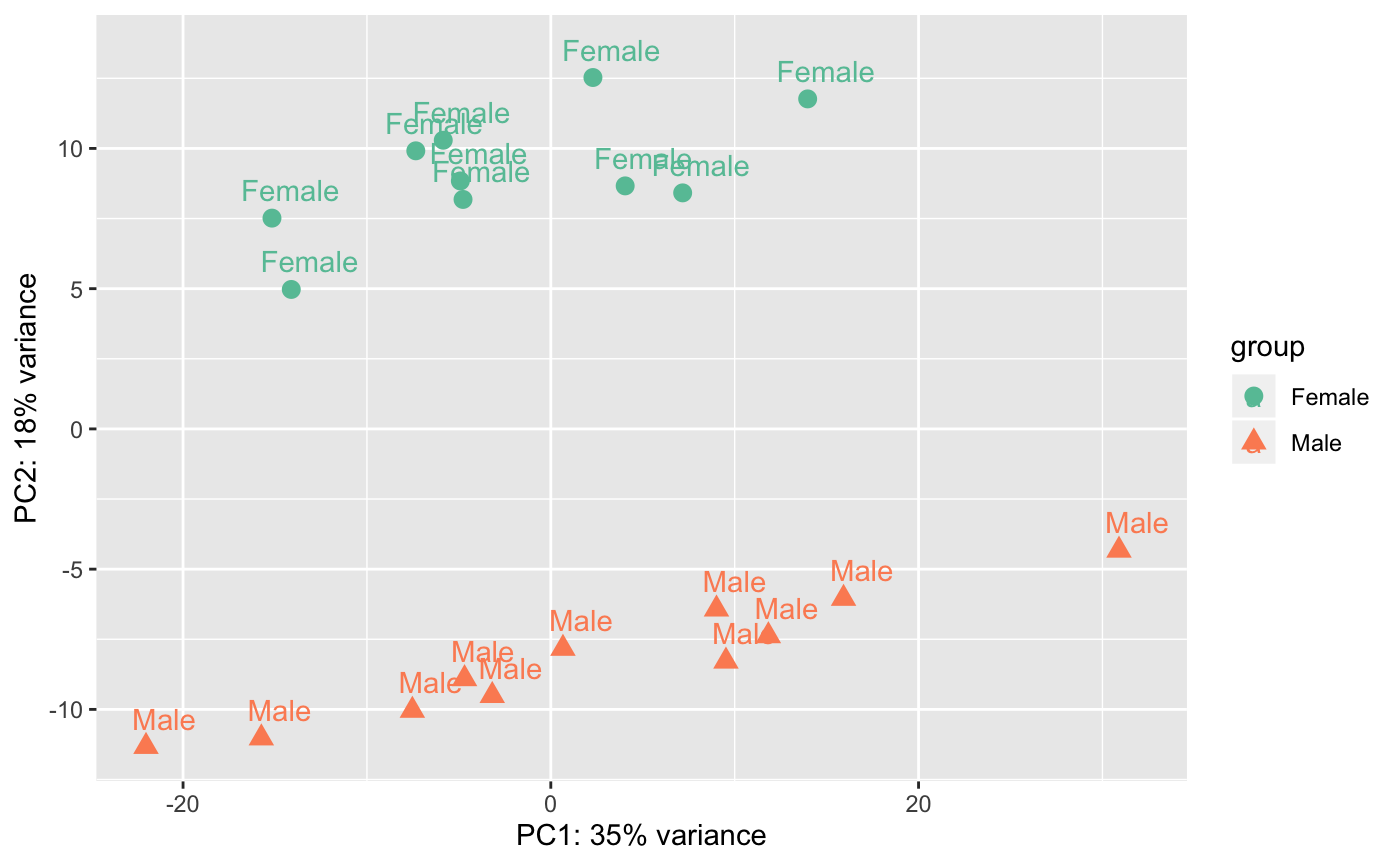nice plot using ggplot2 from prcomp function

degPCA(counts, metadata = NULL, condition = NULL, pc1 = "PC1",
pc2 = "PC2", name = NULL, shape = NULL, data = FALSE)

## Arguments

counts matrix with count data dara.frame with sample information character column in metadata to use to color samples character PC to plot on x-axis character PC to plot on y-axis character if given, column in metadata to print label character if given, column in metadata to shape points Whether return PCA data or just plot the PCA.

## Value

if results <- used, the function return the output of prcomp().

## Examples

data(humanGender)
library(DESeq2)
idx <- c(1:10, 75:85)
dse <- DESeqDataSetFromMatrix(assays(humanGender)[][1:1000, idx],
colData(humanGender)[idx,], design=~group)
degPCA(log2(counts(dse)+0.5), colData(dse),
condition="group", name="group", shape="group")